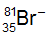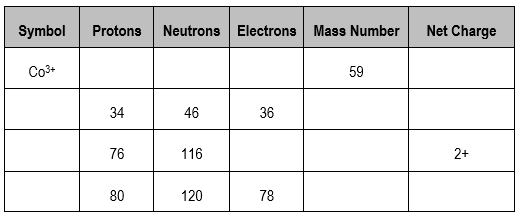Clutch Prep is now a part of Pearson
Ch.2 - Atoms & ElementsWorksheetSee all chapters

# Ions

See all sections
Sections
The Atom
Subatomic Particles
Isotopes
Ions
Atomic Mass
Periodic Table: Classifications
Periodic Table: Group Names
Periodic Table: Representative Elements & Transition Metals
Periodic Table: Element Symbols
Periodic Table: Elemental Forms
Periodic Table: Phases
Periodic Table: Charges
Calculating Molar Mass
Mole Concept
Law of Conservation of Mass
Law of Definite Proportions
Atomic Theory
Law of Multiple Proportions
Millikan Oil Drop Experiment
Rutherford Gold Foil Experiment

Ions represent elements that possess either a positive charge or a negative charge.

###### Determining Ionic Charge

Concept #1: Losing an electron(s) creates a positive charge and gaining an electron(s) creates a negative charge.

Ions are formed from either the loss or gaining of electrons by a neutral element.

Concept #2: A positively charged ion is called a cation and a negatively charged ion is called an anion

A positively charged ion is called a cation and a negatively charged ion is called an anion

Example #1: Determine the number of protons, neutrons, and electrons for the following cation 2713Al+3.

Practice: Give the correct number of protons, neutrons and electrons for the following isotope:.

Practice: In which pair are the two species both isoelectronic and isotopic?

Practice: One isotope of a metallic element has a mass number of 65 and 35 neutrons in the nucleus. The cation that this atom forms has 28 electrons. What is the symbol of the cation?

Practice: Which of the following is the symbol for the ion with a +4 charge, 30 neutrons and 21 electrons?

Practice: Fill in the gaps for the following table.